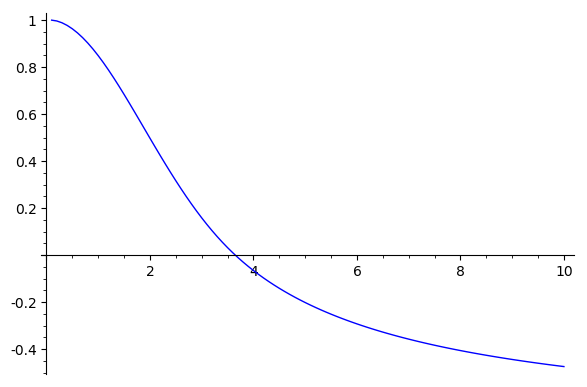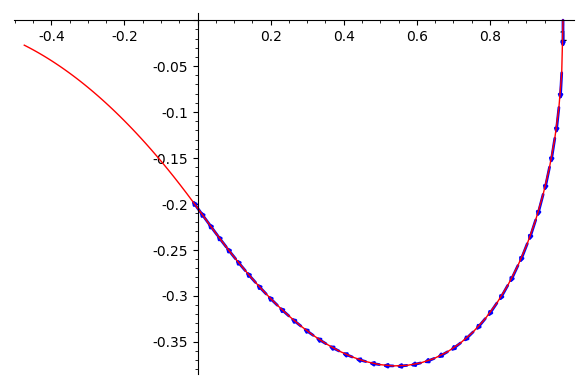Cannot solve differential equation (Lane-Emden equation) numerically

Hi, my friends,

I tried to solve Lane-Emden equation, as model of white dwarf,

$$\frac{d^2x}{dt^2} +\frac2 t \frac{dx}{dt} + x^n = 0, ~~~~ where ~~~~n=\frac 3 2$$

and I have some troubles in sagemath.

I am using following code:

T = ode_solver()
def f_1(t,y): return [y,-2/t*y-y^(3/2)]
T.function = f_1
def j_1(t,y): return [[0, 1], [-3/2*y^(1/2), -2/t], [0,2*y/t^2]]     #Jacobian matrix
T.jacobian = j_1
T.algorithm = "rk8pd"
T.ode_solve(y_0=[1,0], t_span=[0,10], num_points=1000)
f = T.interpolate_solution()
plot(f, 0, 10)

Above code is very similar of the example in sagemath reference: Van der Pol equation

Both equations (Lane-Emden and Van der Pol) are non-linear differential equation, therefore, are not easy to solve.

I don't know where comes to problem in above codes, can someone give me a help?

John

edit retag close merge delete

Sort by » oldest newest most votedI don't know how to fix that code, but as an alternative you can use desolve_system_rk4:

var('y0 y1 t')
ode_rhs = [y1, -2/t*y1 - y0^(3/2)]
points = desolve_system_rk4(ode_rhs,[y0,y1],ics=[0.1,1,0],ivar=t,end_points=10,step=0.1)

To plot y0 (i.e. $x$) against t:

ty0_points = [ [i,j] for i,j,k in points]
list_plot(ty0_points, plotjoined=True)To plot the curve in the (y0,y1)-plane (together with the vector field):

y0y1_points = [ [j,k] for i,j,k in points]
list_plot(y0y1_points, plotjoined=True, color='red') + sum(arrow2d(p[1:], vector(p[1:]) + vector([eqn_rhs.subs({t : p, y0 : p, y1: p}) for eqn_rhs in ode_rhs]).normalized()*0.03,arrowsize=1,color='blue') for p in points if p >= 0)The vector field only makes sense when y0 >= 0 (same for the ODE and its "solution" plotted above). I don't know what the solver does past that point; I wouldn't trust it.

more

Thank you very muchfor you nice answer, Mr. Buring. For the first plot, is there a simple way to find t where y0=0?

You're welcome. You can do min(ty0_points,key=lambda (t,y0): abs(y0)) to find a pair $(t,y_0)$ where $y_0$ is minimal in absolute value. To get a better approximation, you can make the step size smaller.